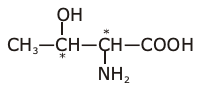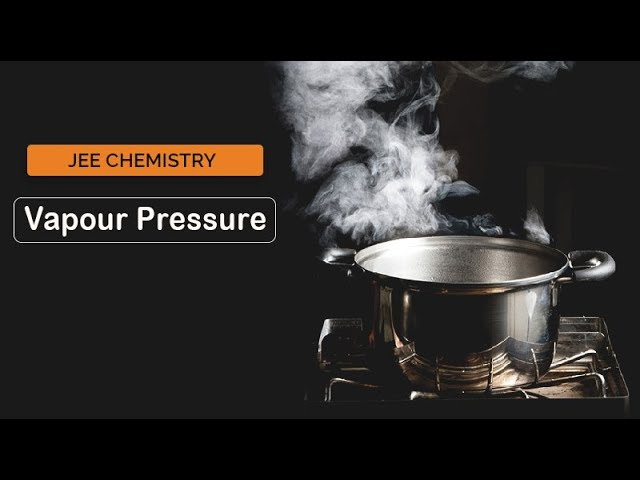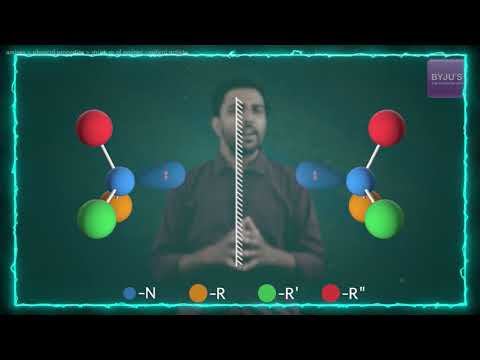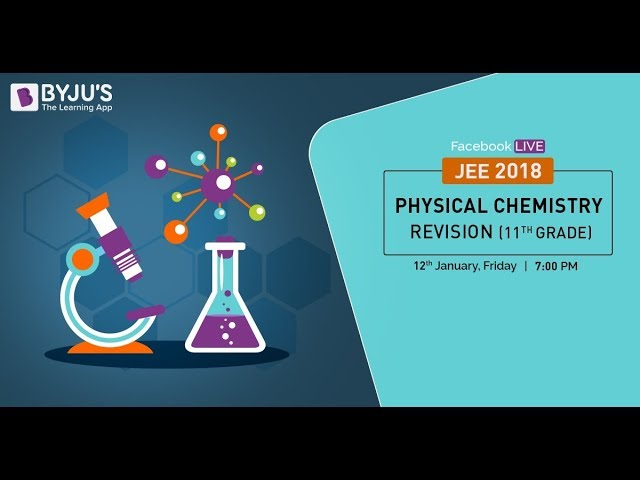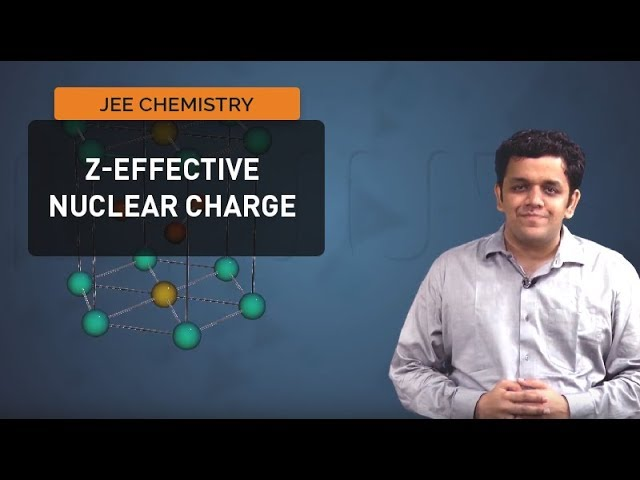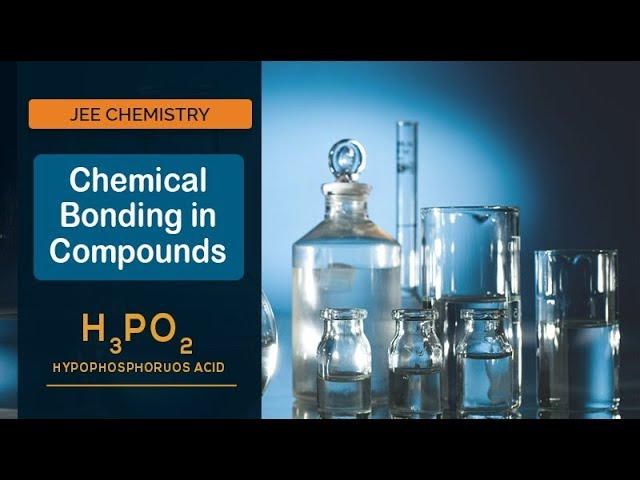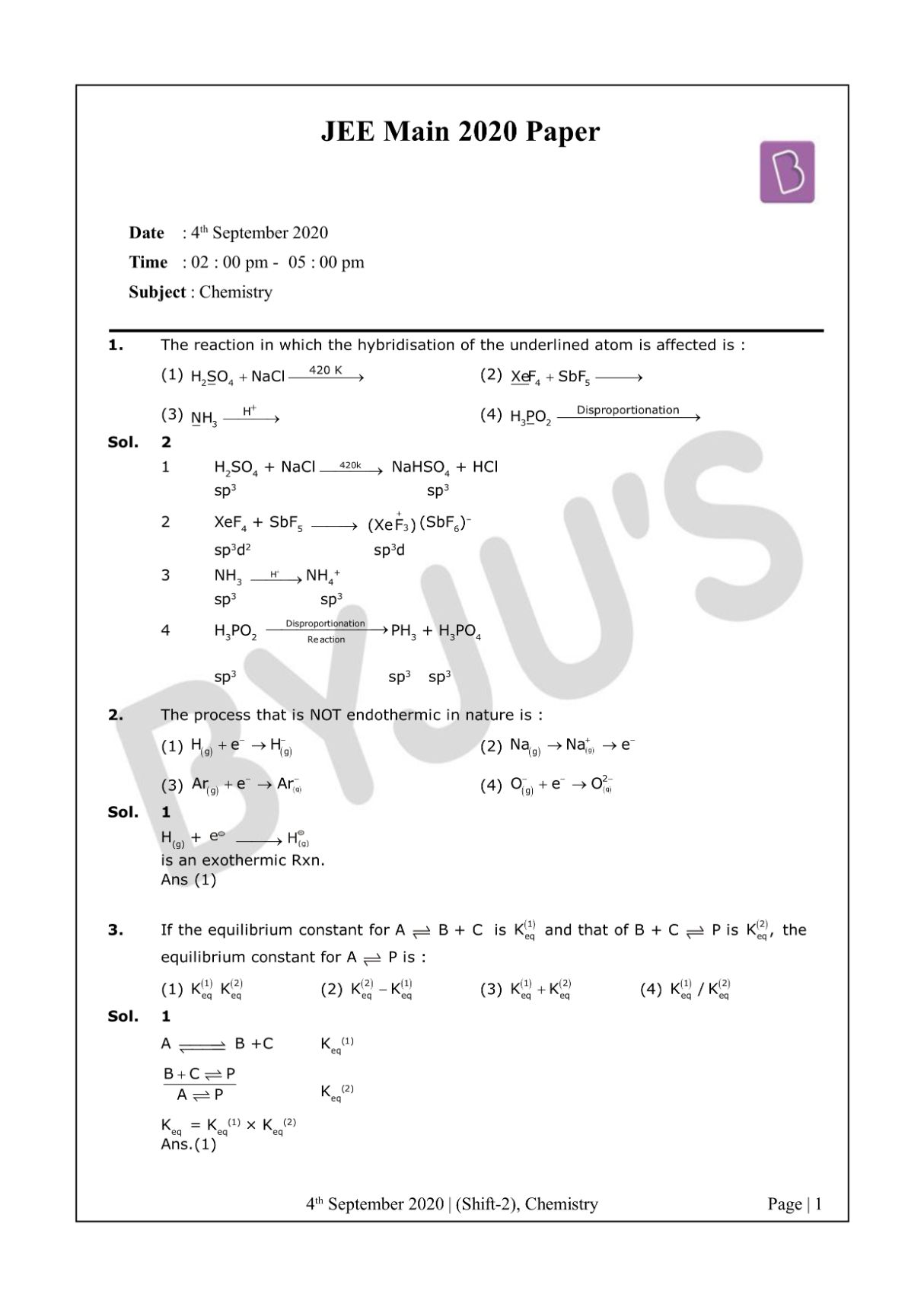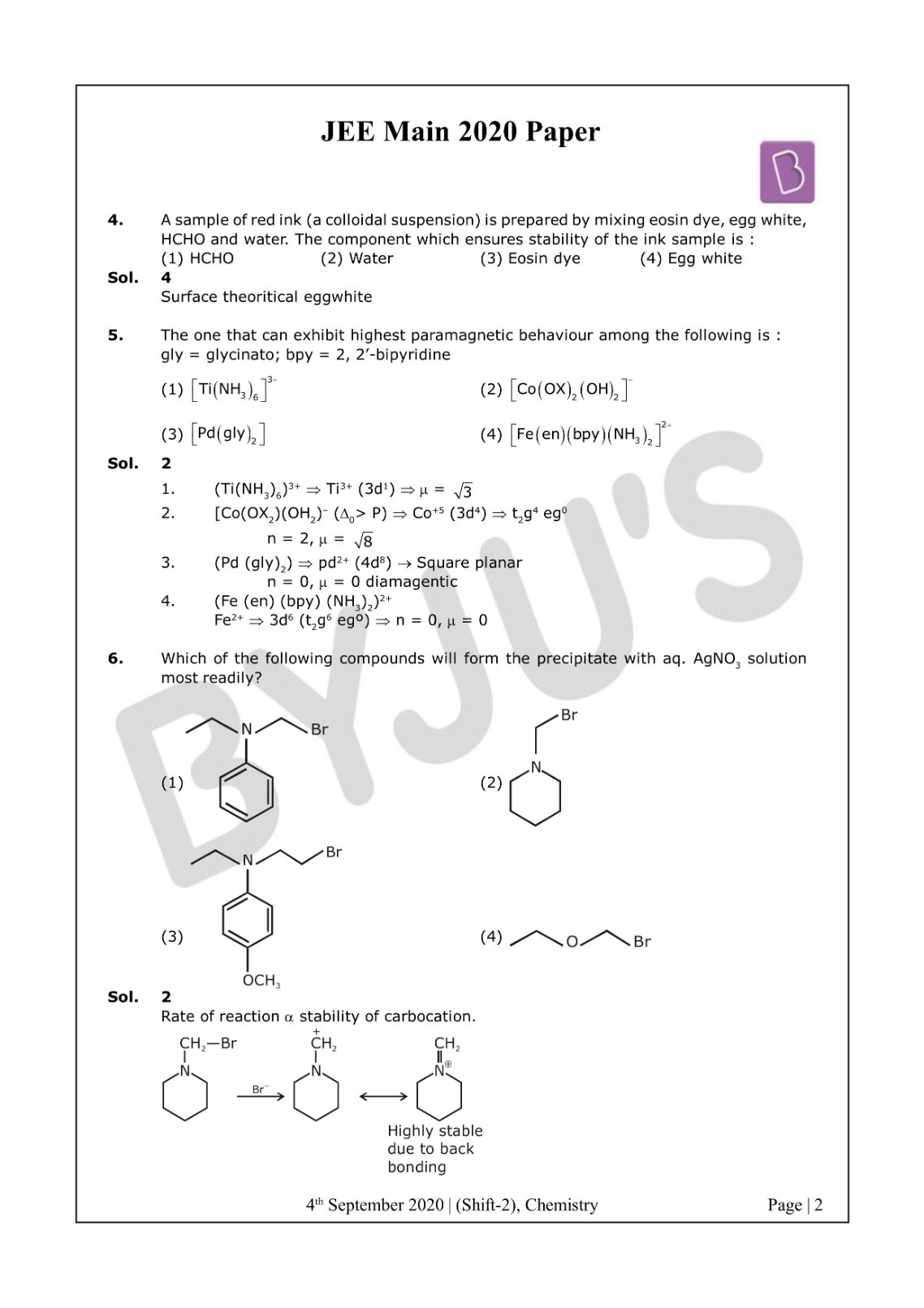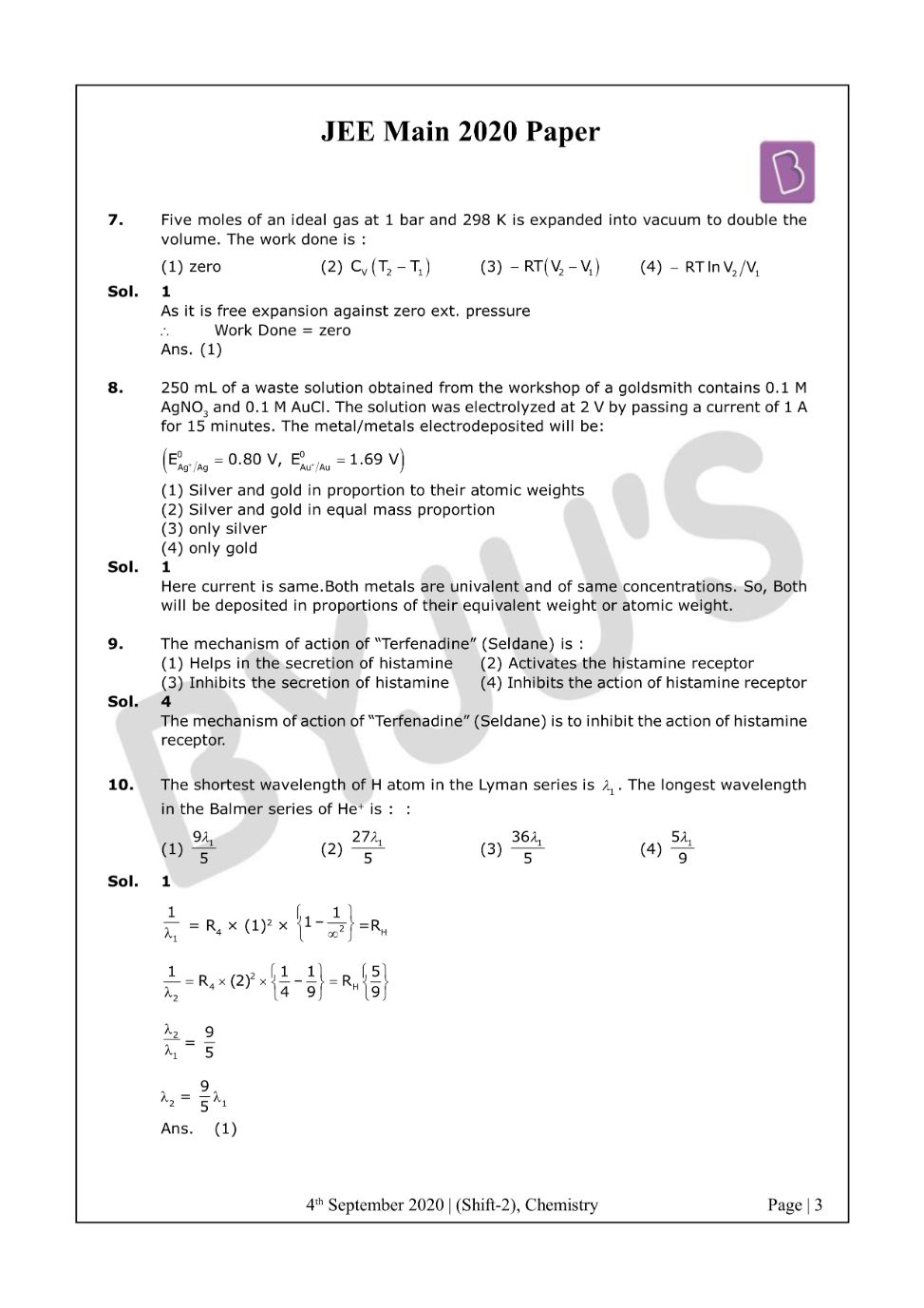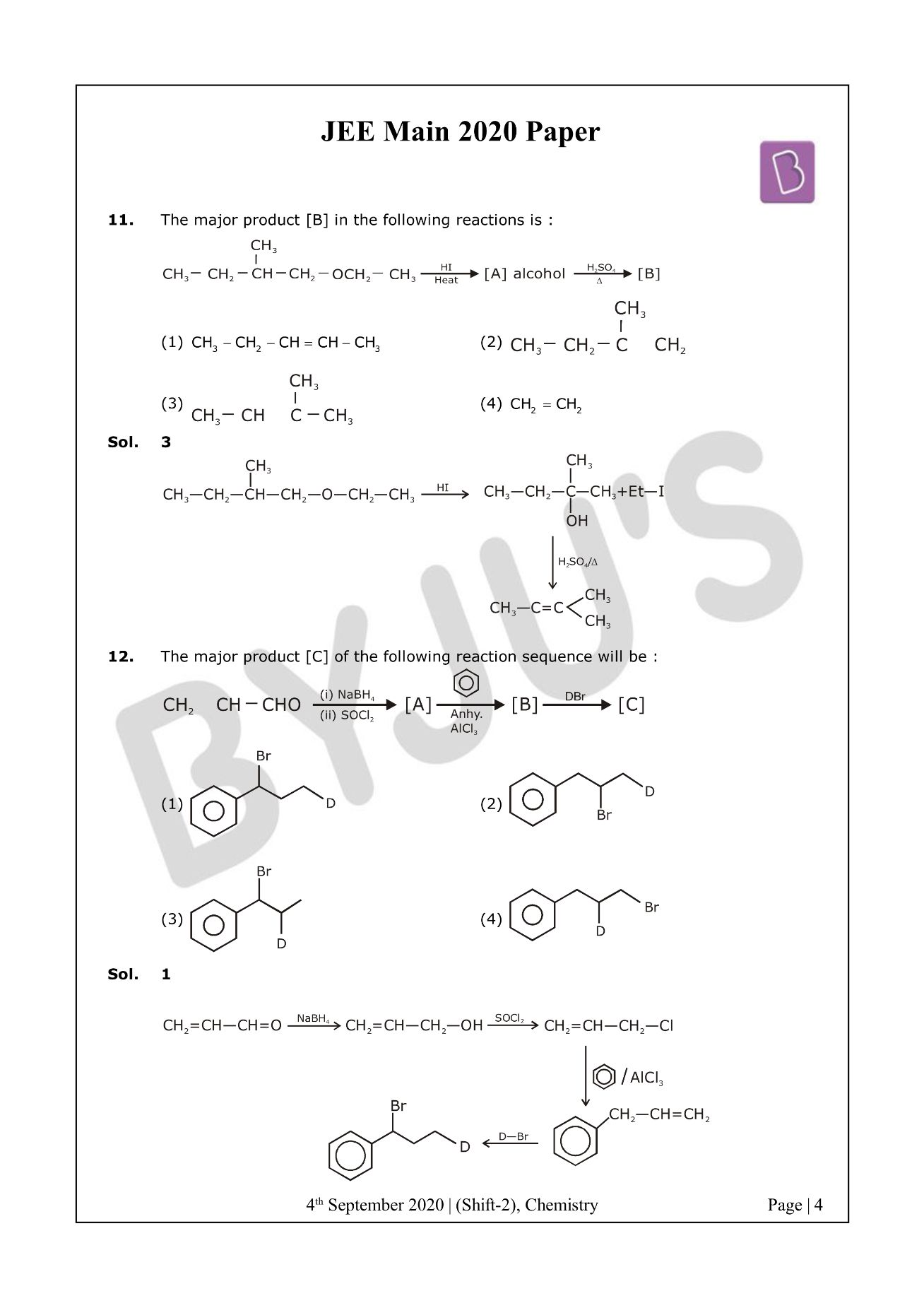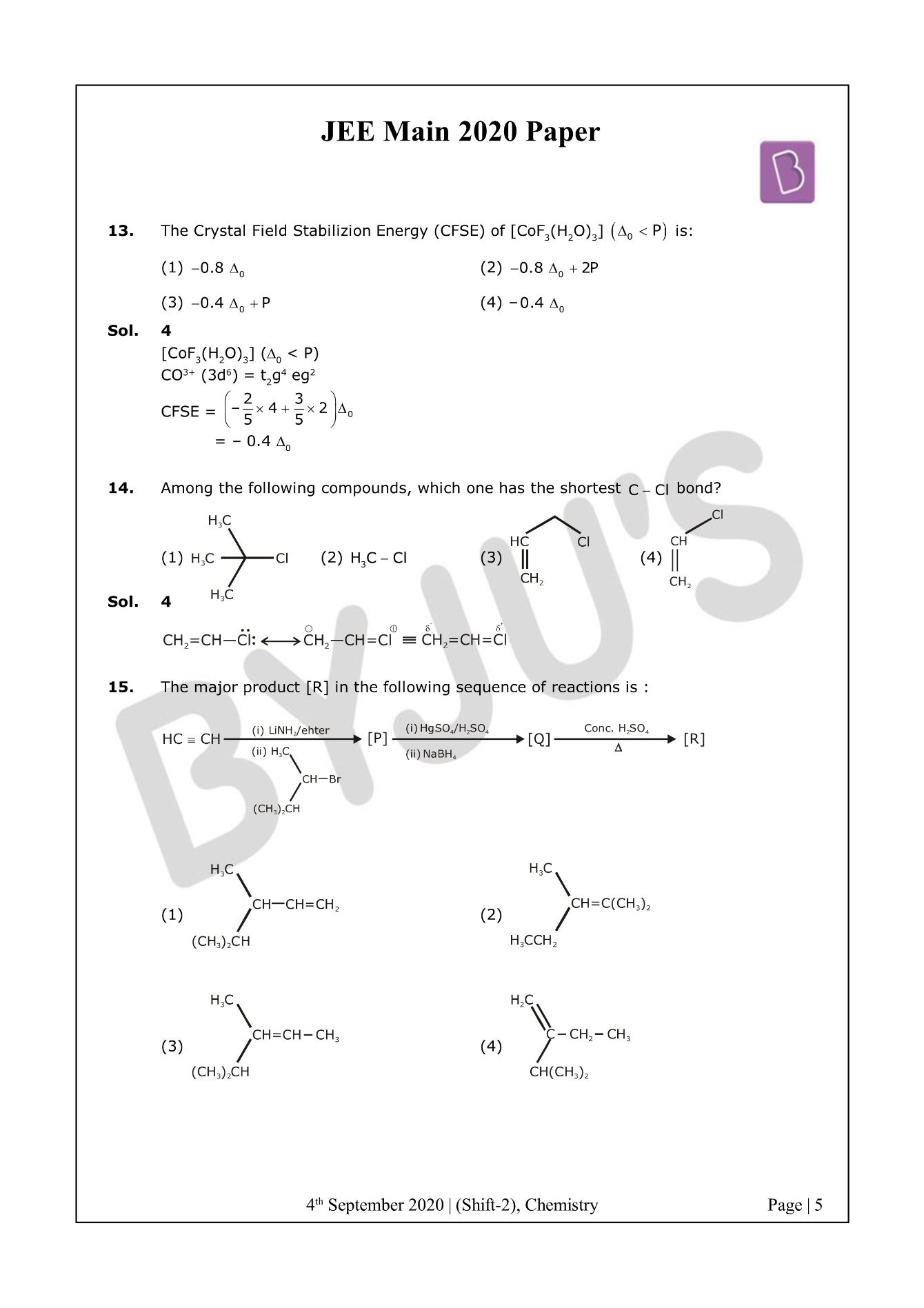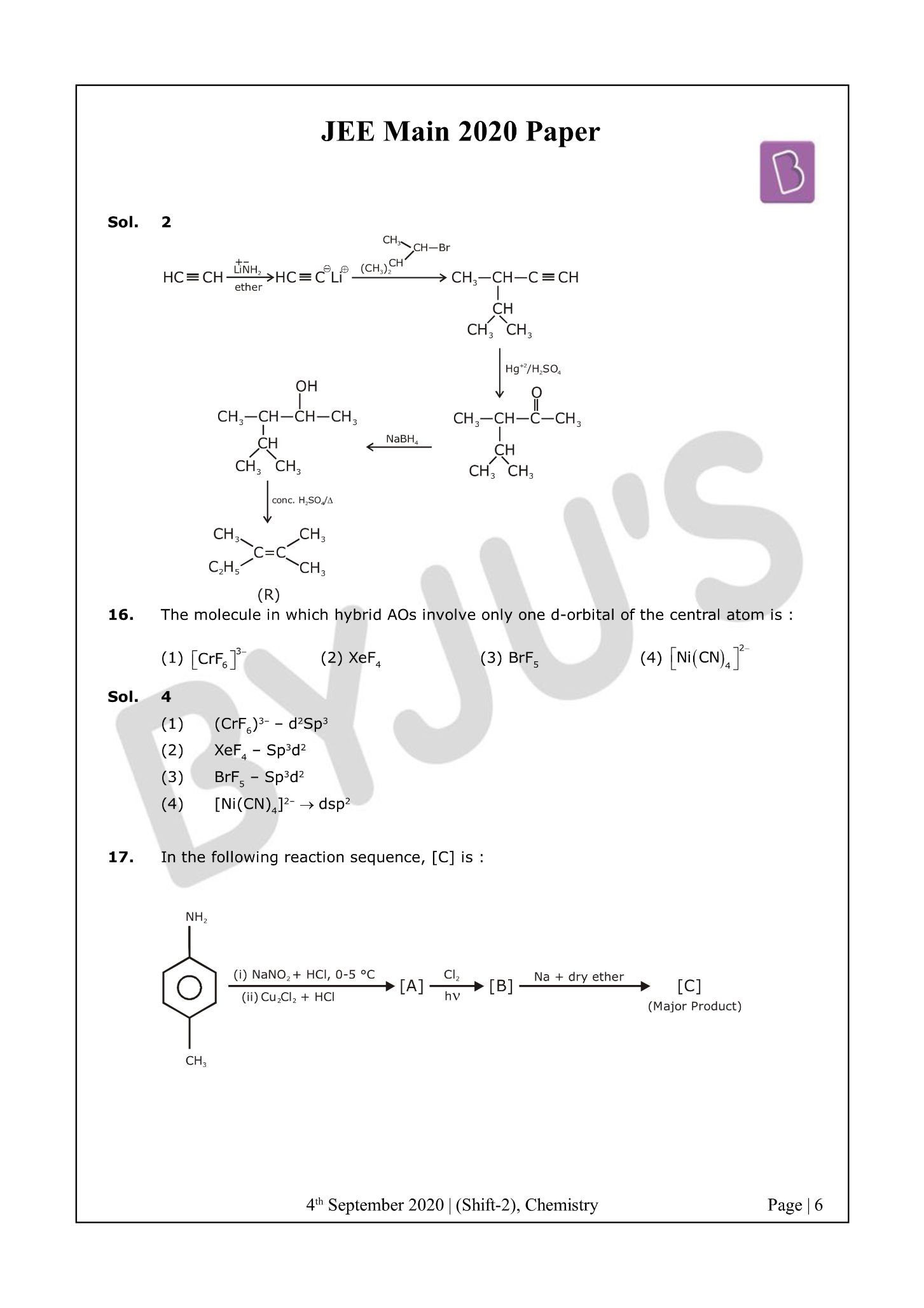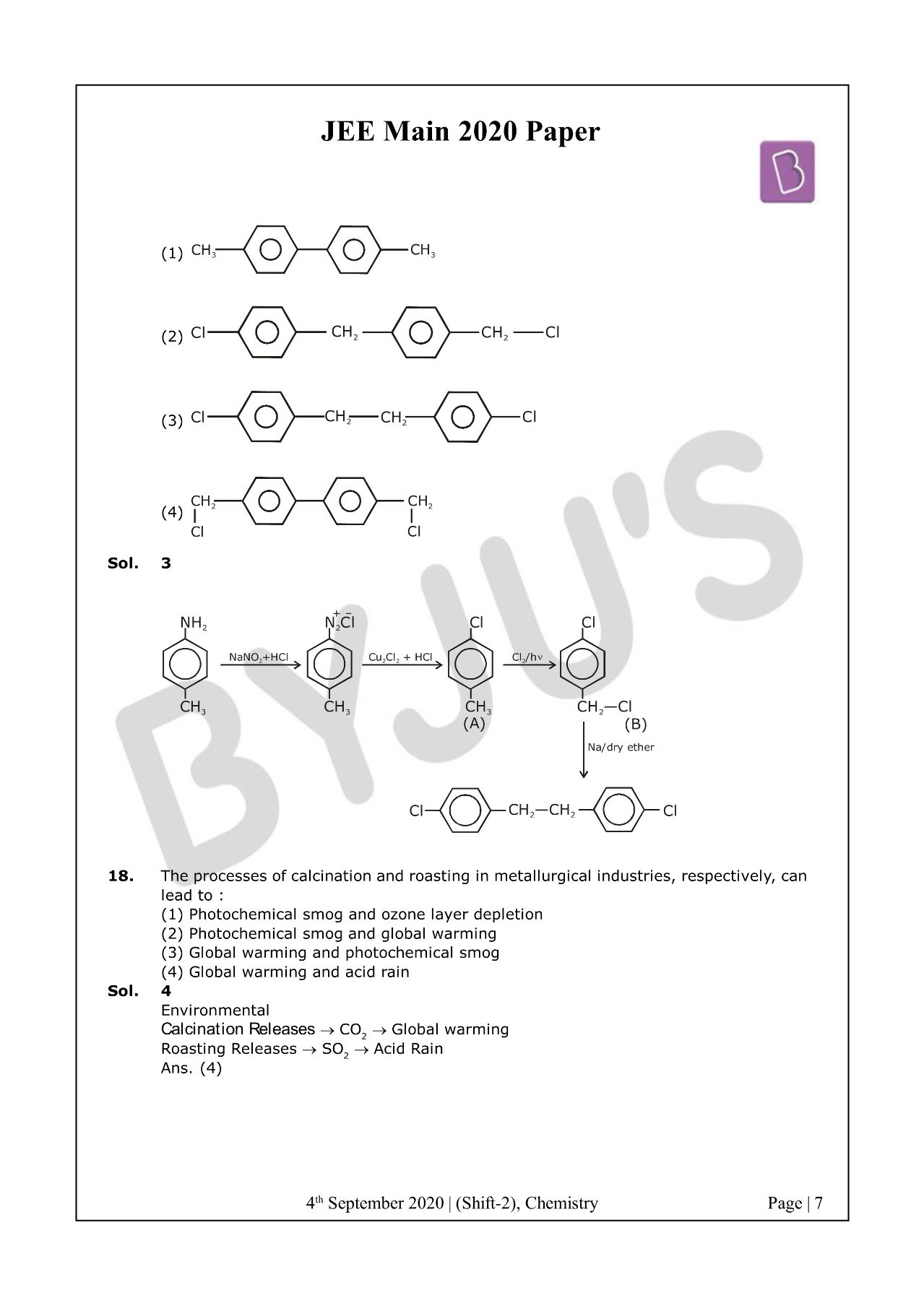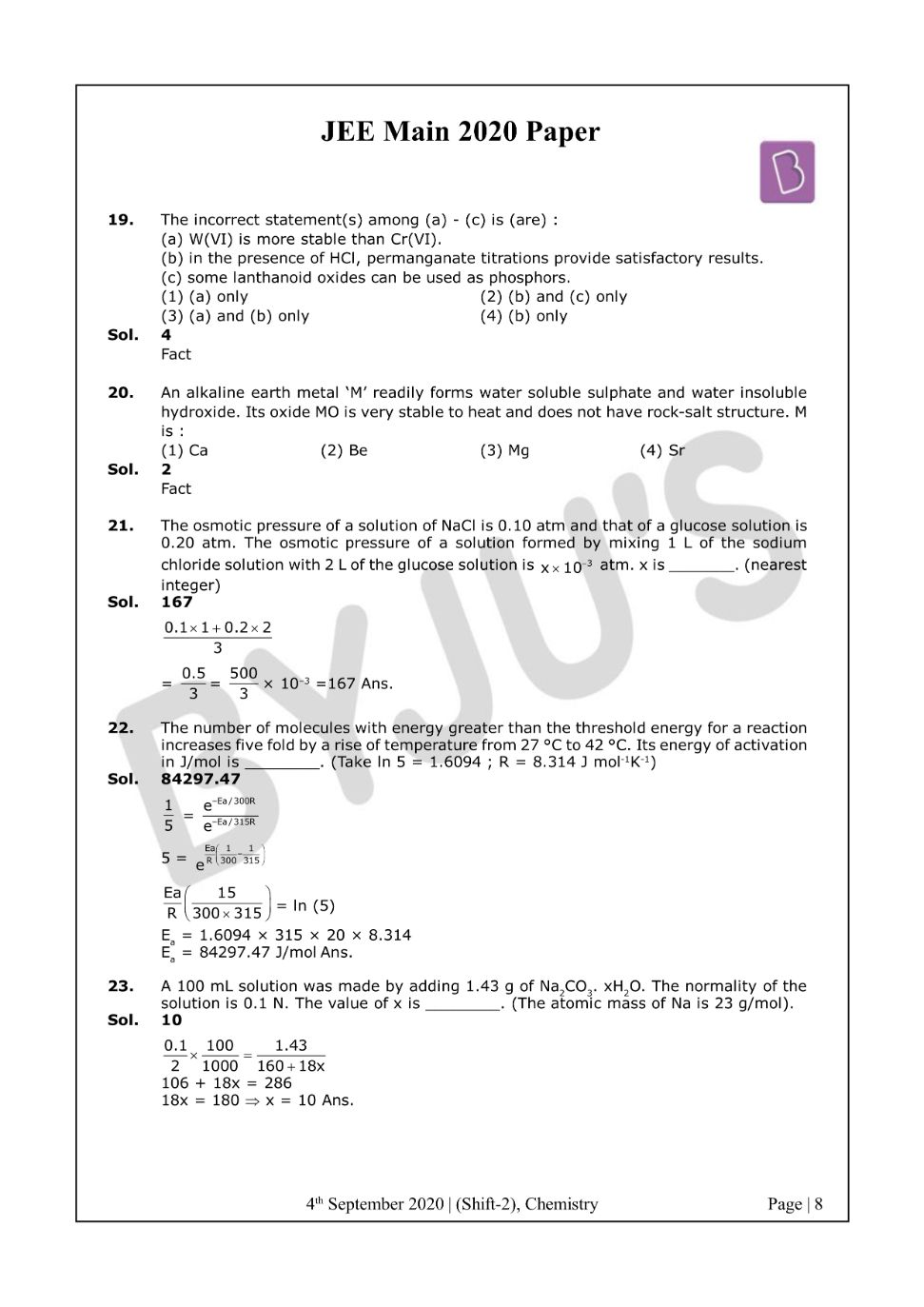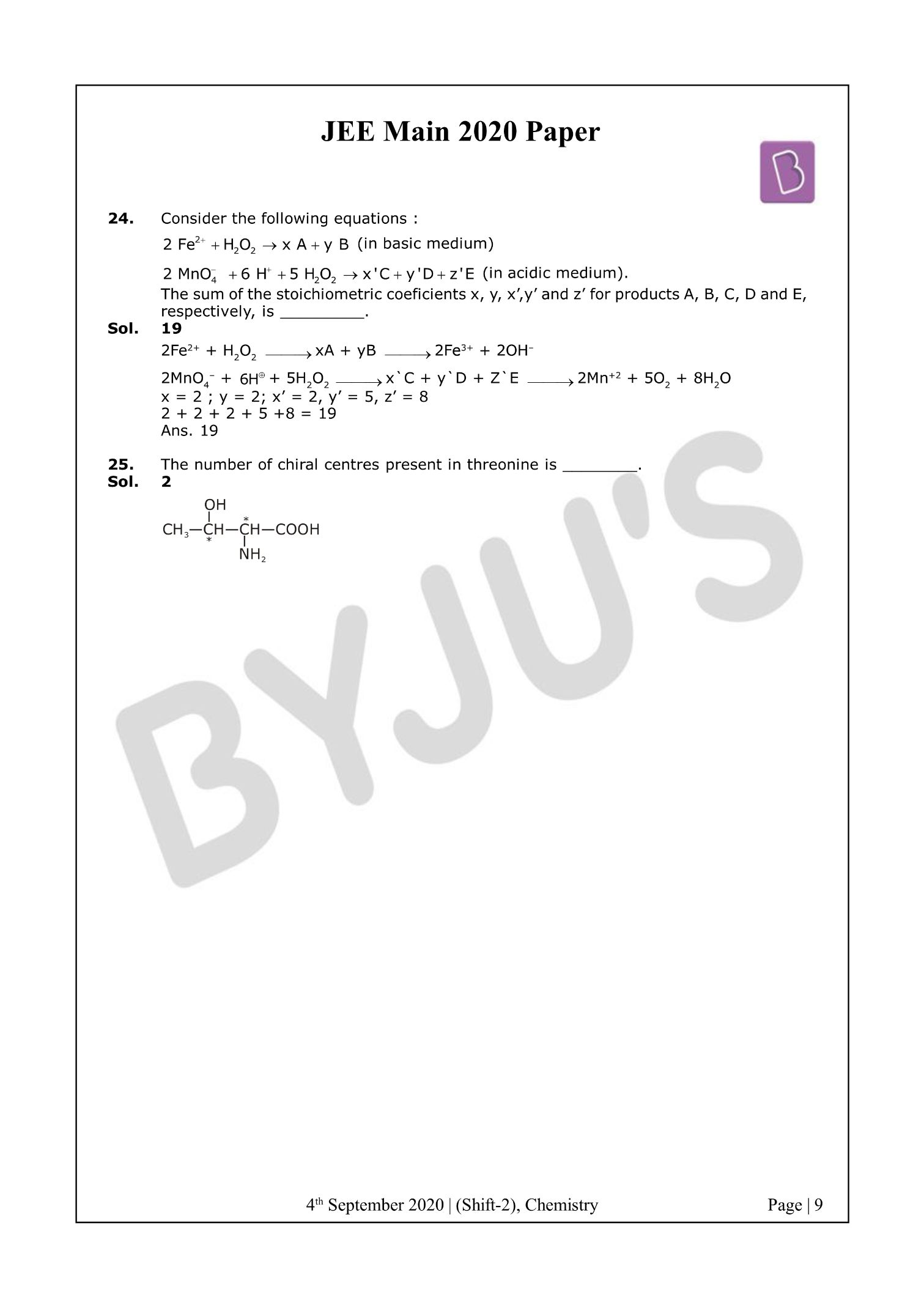Win up to 100% scholarship on Aakash BYJU'S JEE/NEET courses with ABNAT Win up to 100% scholarship on Aakash BYJU'S JEE/NEET courses with ABNAT

# JEE Main 2020 Chemistry Paper With Solutions Sept 4 (Shift 2)

JEE Main 2020 Paper with Solutions Chemistry – Shift 2 is a perfect resource for the candidates who are appearing for JEE Main to have an idea of the difficulty level of the exam. The answer keys are prepared in such a way that the candidates can have a crystal clear understanding of the JEE Main question paper.
The solutions uploaded at BYJU’S will enable the students to obtain a fair idea about the distribution of marks with respect to each topic. Practising these questions will help them understand the amount of preparation required to face the examination.
September 4 Shift 2 – Chemistry
Question 1: The reaction in which the hybridisation of the underlined atom is affected is: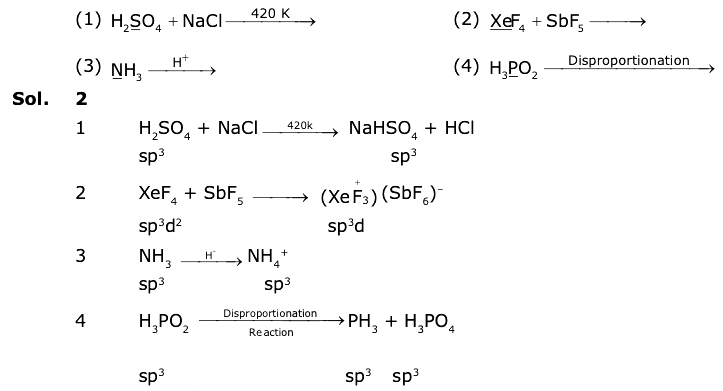Question 2: The process that is NOT endothermic in nature is :
1) H(g) + e ? H(g)
2) Na(g) ? Na(g) ? e
3) Ar(g) + e ? Ar(g)
4) O(g) + e ? O(g)2-

H(g) + e ? H(g) is an exothermic reaction.

Question 3: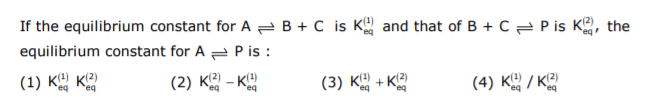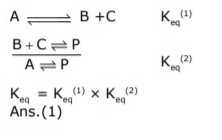Question 4: A sample of red ink (a colloidal suspension) is prepared by mixing eosin dye, egg white, HCHO and water. The component which ensures the stability of the ink sample is :
1) HCHO
2) Water
3) Eosin dye
4) Egg white

Surface theoretical egg white

Question 5: The one that can exhibit the highest paramagnetic behaviour among the following is: gly = glycinato; bpy = 2, 2’-bipyridine
1) [Ti (NH3)6]3+
2) [Co (OX)2 (OH)2]
3) [Pd (gly)2]
4) [Fe (en) (bpy) (NH3)2]2+

 [Ti (NH3)6]3+ ? Ti3+ (3d1) ? ?? = v3

 [Co (OX)2 (OH)2] (?0 > P) ? Co+5 (3d4) ? t2g4 eg0

 [Pd (gly)2] ? pd2+ (4d8) ? Square planar

n = 0, ?? = 0, diamagnetic

 [Fe (en) (bpy) (NH3)2]2+

Fe2+ ? 3d6 (t2g6 eg0) ? n = 0, ?? = 0

Question 6: Which of the following compounds will form the precipitate with aq. AgNO3 solution most readily?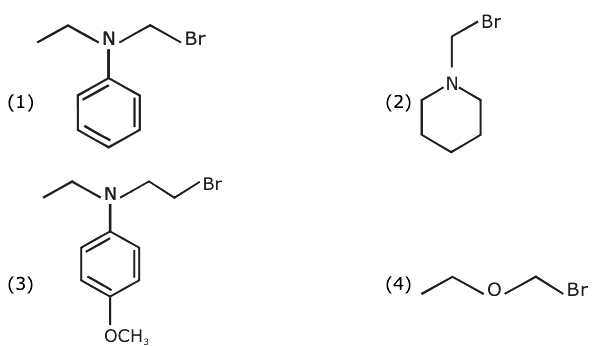Rate of reaction ? stability of carbocation.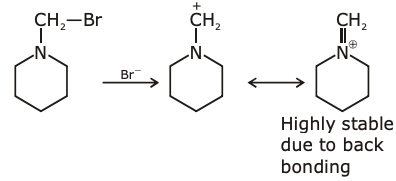Question 7: Five moles of an ideal gas at 1 bar and 298 K is expanded into the vacuum to double the volume. The work done is:
1) zero
2) Cv [T2 – T1]
3) – RT (V2 – V1)
4) – RT ln (V2/V1)

As it is free expansion against zero external pressure, work done = zero.

Question 8: 250 mL of a waste solution obtained from the workshop of a goldsmith contains 0.1 M AgNO3 and 0.1 M AuCl. The solution was electrolyzed at 2 V by passing a current of 1 A for 15 minutes. The metal/metals electrodeposited will be: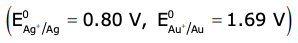1) Silver and gold in proportion to their atomic weights
2) Silver and gold in equal mass proportion
3) only silver
4) only gold

Here the current is the same. Both metals are univalent and of the same concentrations. So, both will be deposited in proportions of their equivalent weight or atomic weight.

Question 9: The mechanism of action of “Terfenadine” (Seldane) is:
1) Helps in the secretion of histamine
2) Activates the histamine receptor
3) Inhibits the secretion of histamine
4) Inhibits the action of histamine receptor

The mechanism of action of “Terfenadine” (Seldane) is to inhibit the action of histamine receptors.

Question 10: The shortest wavelength of the H atom in the Lyman series is ?1. The longest wavelength in the Balmer series of He+ is:
1) 9?1/5
2) 27?1/5
3) 36?1/5
4) 5?1/9

(1/?1) = R4 × (1)2 × {1 – [1/82]} = RH

(1/?2) = R4 × (2)2 × {[1/4] – [1/9]} = RH {5/9}

(?2/?1) = 9/5

?2 = (9/5) ?1

Question 11: The major product [B] in the following reactions is: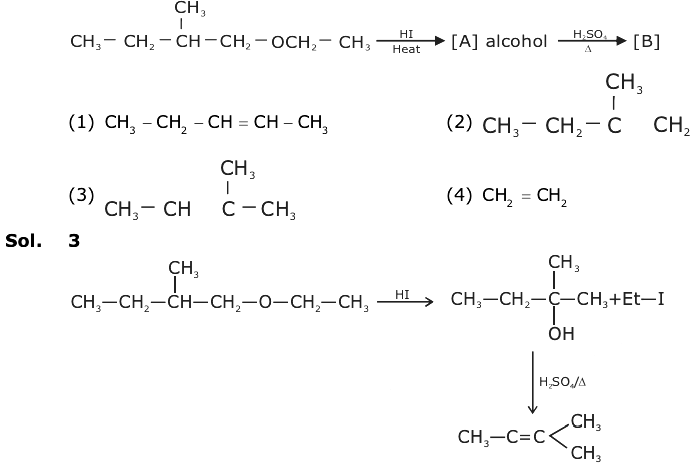Question 12: The major product [C] of the following reaction sequence will be: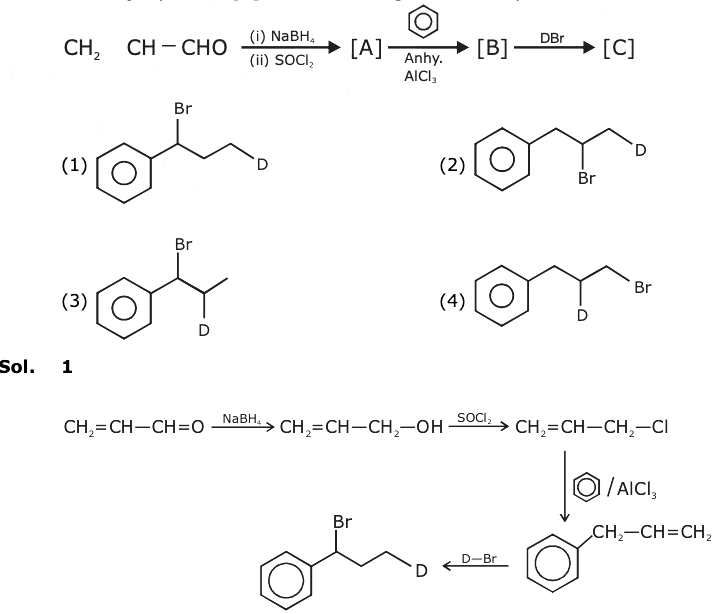Question 13: The Crystal Field Stabilization Energy (CFSE) of [CoF3(H2O)3] (?0 < P) is:
1) – 0.8?0
2) – 0.8?0 + 2P
3) – 0.4?0 + P
4) – 0.4?0

[CoF3(H2O)3] (?0 < P)

CO3 + (3d6) = t2 g4 eg2

CFSE = [(-2/5) * 4 + (3/5) * 2] ?0

= – 0.4 ?0

Question 14: Among the following compounds, which one has the shortest C – Cl bond?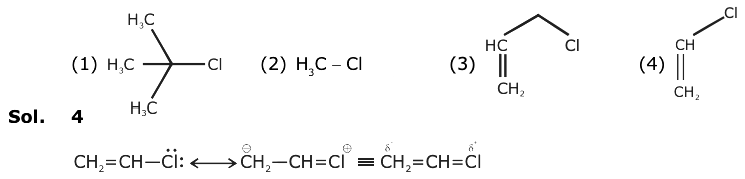Question 15: The major product [R] in the following sequence of reactions is: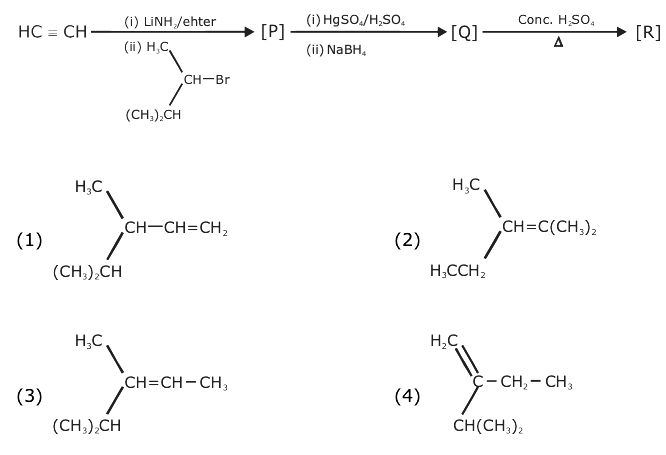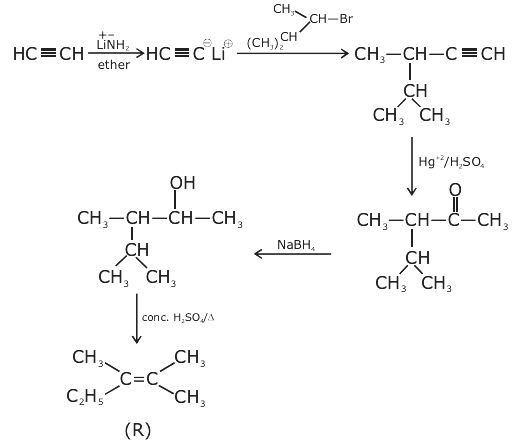Question 16: The molecule in which hybrid AOs involve only one d-orbital of the central atom is:
1) [CrF6]3-
2) XeF4
3) BrF5
4) [Ni (CN)4]2-

(1) (CrF6)3– – d2Sp3

(2) XeF4 – Sp3d2

(3) BrF5 – Sp3d2

(4) [Ni(CN)4]2– ? dsp2

Question 17: In the following reaction sequence, [C] is: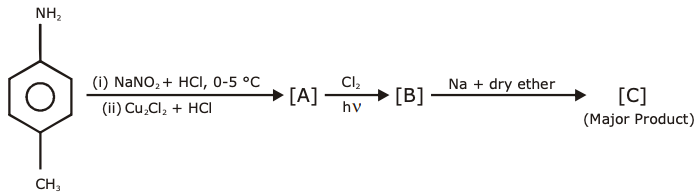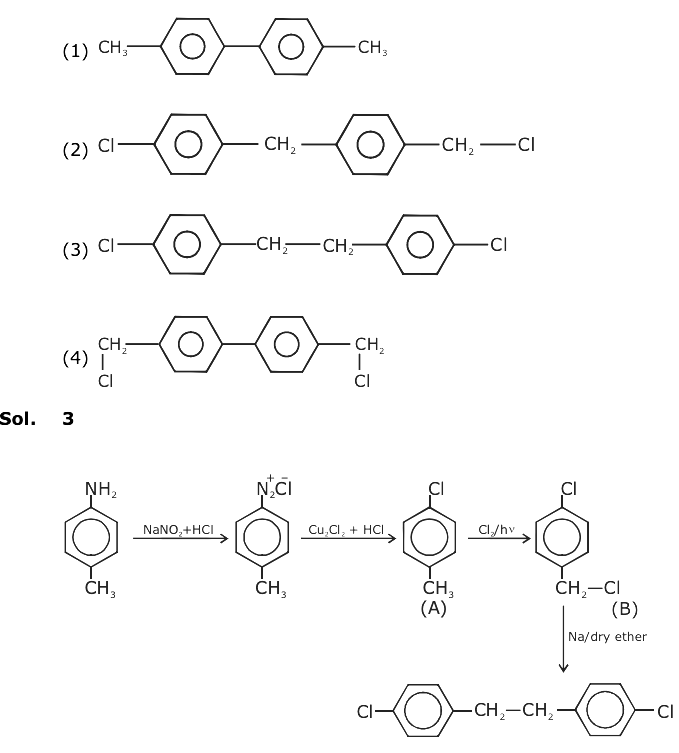Question 18: The processes of calcination and roasting in metallurgical industries, respectively, can lead to:
1) Photochemical smog and ozone layer depletion
2) Photochemical smog and global warming
3) Global warming and photochemical smog
4) Global warming and acid rain

Environmental

Calcination Releases ? CO2 ? Global warming

Roasting Releases ? SO2 ? Acid Rain

Question 19: The incorrect statement(s) among (a) – (c) is (are):

(a) W(VI) is more stable than Cr(VI).

(b) in the presence of HCl, permanganate titrations provide satisfactory results.

(c) some lanthanoid oxides can be used as phosphors.

1) (a) only
2) (b) and (c) only
3) (a) and (b) only
4) (b) only

Fact

Question 20: An alkaline earth metal ‘M’ readily forms water-soluble sulphate and water-insoluble hydroxide. Its oxide MO is very stable to heat and does not have a rock-salt structure. M is :
1) Ca
2) Be
3) Mg
4) Sr

Fact

Question 21: The osmotic pressure of a solution of NaCl is 0.10 atm and that of a glucose solution is 0.20 atm. The osmotic pressure of a solution formed by mixing 1 L of the sodium chloride solution with 2 L of the glucose solution is x × 10-3 atm. x is _______. (nearest integer)

[0.1 × 1 + 0.2 × 2]/3 = 0.5/3 = (500/3) × 10-3 = 167

Question 22: The number of molecules with energy greater than the threshold energy for a reaction increases fivefold by a rise of temperature from 27°C to 42 °C. Its energy of activation in J/mol is ________. (Take ln 5 = 1.6094 ; R = 8.314 J mol-1K-1)

(1/5) = e-Ea/300R/e-Ea/315R

(5) = e[Ea/R][(1/300) – (1/315)]

(Ea/R) [(15/(300 × 315)] = ln 5

Ea = 1.6094 × 315 × 20 × 8.314

Ea = 84297.47 J/mol

Question 23: A 100 mL solution was made by adding 1.43 g of Na2CO3. xH2O. The normality of the solution is 0.1 N. The value of x is ________. (The atomic mass of Na is 23 g/mol).

(0.1/2) × (100/1000) = (1.43/[160 + 18x])

106 + 18x = 286

18x = 180

x = 10

Question 24: Consider the following equations:

2 Fe2+ + H2O2 ? x A + y B (in basic medium)

2 MnO4 + 6 H+ + 5 H2O2 ? x ‘C + y ‘D + z’E (in acidic medium).

The sum of the stoichiometric coefficients x, y, x’,y’ and z’ for products A, B, C, D and E, respectively, is _________.

2Fe2+ + H2O2 ? xA + yB ? 2Fe3+ + 2OH

2MnO4 + 6H+ + 5H2O2 ? xC + yD + Z`E ? 2Mn+2 + 5O2 + 8H2O

x = 2 ; y = 2; x’ = 2, y’ = 5, z’ = 8

2 + 2 + 2 + 5 + 8 = 19

Question 25: The number of chiral centres present in threonine is ________.